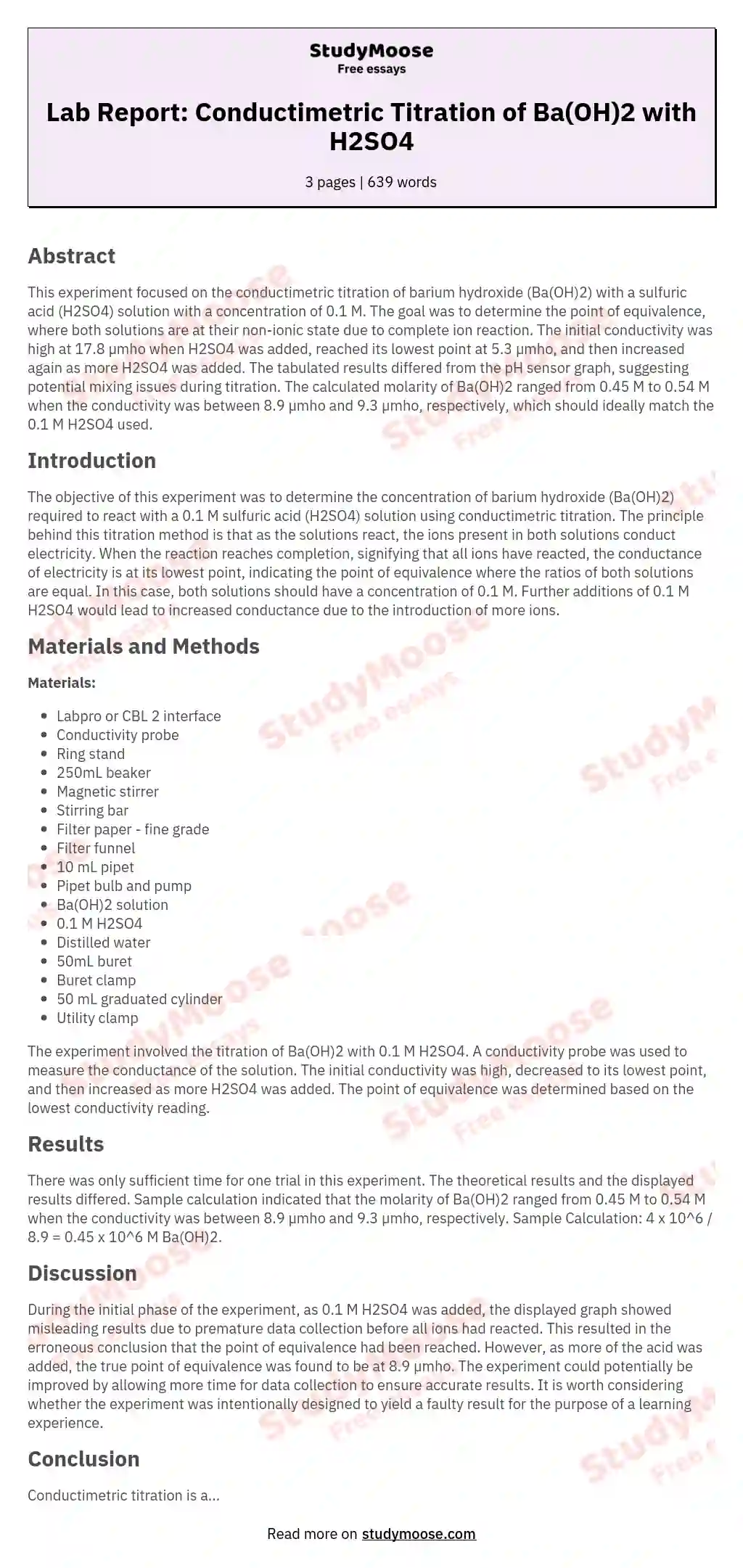# Lab Report: Conductimetric Titration of Ba(OH)2 with H2SO4

Categories: Titration

## Abstract

This experiment focused on the conductimetric titration of barium hydroxide (Ba(OH)2) with a sulfuric acid (H2SO4) solution with a concentration of 0.1 M. The goal was to determine the point of equivalence, where both solutions are at their non-ionic state due to complete ion reaction. The initial conductivity was high at 17.8 µmho when H2SO4 was added, reached its lowest point at 5.3 µmho, and then increased again as more H2SO4 was added. The tabulated results differed from the pH sensor graph, suggesting potential mixing issues during titration.

The calculated molarity of Ba(OH)2 ranged from 0.45 M to 0.54 M when the conductivity was between 8.9 µmho and 9.3 µmho, respectively, which should ideally match the 0.1 M H2SO4 used.

## Introduction

The objective of this experiment was to determine the concentration of barium hydroxide (Ba(OH)2) required to react with a 0.1 M sulfuric acid (H2SO4) solution using conductimetric titration. The principle behind this titration method is that as the solutions react, the ions present in both solutions conduct electricity.

Get quality help nowProf. FinchVerified writer

Proficient in: Titration4.7 (346)

“ This writer never make an mistake for me always deliver long before due date. Am telling you man this writer is absolutely the best. ”+84 relevant experts are online

When the reaction reaches completion, signifying that all ions have reacted, the conductance of electricity is at its lowest point, indicating the point of equivalence where the ratios of both solutions are equal. In this case, both solutions should have a concentration of 0.1 M. Further additions of 0.1 M H2SO4 would lead to increased conductance due to the introduction of more ions.

## Materials and Methods

Materials:

• Labpro or CBL 2 interface
• Conductivity probe
• Ring stand
• 250mL beaker
• Magnetic stirrer
• Stirring bar
• Filter paper - fine grade
• Filter funnel
• 10 mL pipet
• Pipet bulb and pump
• Ba(OH)2 solution
• 0.1 M H2SO4
• Distilled water
• 50mL buret
• Buret clamp
• Utility clamp

The experiment involved the titration of Ba(OH)2 with 0.1 M H2SO4. A conductivity probe was used to measure the conductance of the solution. The initial conductivity was high, decreased to its lowest point, and then increased as more H2SO4 was added. The point of equivalence was determined based on the lowest conductivity reading.

## Results

There was only sufficient time for one trial in this experiment. The theoretical results and the displayed results differed. Sample calculation indicated that the molarity of Ba(OH)2 ranged from 0.45 M to 0.54 M when the conductivity was between 8.9 µmho and 9.3 µmho, respectively.

Sample Calculation: 4 x 10^6 / 8.9 = 0.45 x 10^6 M Ba(OH)2.

## Discussion

During the initial phase of the experiment, as 0.1 M H2SO4 was added, the displayed graph showed misleading results due to premature data collection before all ions had reacted. This resulted in the erroneous conclusion that the point of equivalence had been reached. However, as more of the acid was added, the true point of equivalence was found to be at 8.9 µmho. The experiment could potentially be improved by allowing more time for data collection to ensure accurate results.

It is worth considering whether the experiment was intentionally designed to yield a faulty result for the purpose of a learning experience.

## Conclusion

Conductimetric titration is a viable method for determining the concentration of an unknown solution. However, it requires patience to ensure that ionization results are obtained at the equimolar concentration of both solutions. In this experiment, an error was evident as the 0.1 M H2SO4 did not achieve equimolarity at 1 mL, as expected based on the conductivity readings. The true point of equivalence was reached at 4 mL of 0.1 M H2SO4 with a conductivity of 8.9 µmho, as displayed on the pH sensor graph. The error highlights the importance of careful data collection and patience in conducting titrations.

## Recommendations

For future experiments of this nature, it is advisable to ensure thorough mixing of solutions during titration to avoid misleading results. Additionally, sufficient time should be allocated for data collection to accurately determine the point of equivalence. Further investigations may also be warranted to explore the discrepancies between theoretical and displayed results in greater detail.